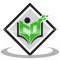## What is the importance of the periodic table?Updated on 10-Oct-2022 09:44:39
The Periodic Table provides the names, atomic numbers, symbols and atomic weights of known elements. It serves as a great tool for solving chemistry problems.A periodic table is divided into groups (columns), where elements with each group behave similarly while bonding with other elements; and periods (rows), where elements in one period have the same number of electron shells. The periodic table is important because it is organized to provide a great deal of information about elements and how they relate to one another in one reference. The table can be used to predict the properties of all the elements, ... Read More

## Answer the following questions-1. Which parts of the banana plant are edible? 2. Which is the edible part of the tomato?Updated on 26-Apr-2023 09:32:30
1. The parts of the banana that are edible are fruit and the flower. 2. The parts of the banana that are edible are the fruit itself, which means every part of the tomato is edible even it's seeds.

## What is Photosynthes?Updated on 26-Apr-2023 09:32:50
Photosynthesis is a process by which plants make their own food in the presence of sunlight, carbon dioxide, water, minerals and chlorophyll present in leaves. Carbon Dioxide + Water + Sunlight ------>       Glucose    + Oxygen                                                                       (Carbohydrate) Process: 1) Plants take up carbon dioxide from the atmosphere through the tiny pores known as stomata present on the surface of the leaves. 2) Plants absorb the water and minerals from the soil with the help of roots and transport them to the leaves of the plant where the synthesis of food takes place. 3) The green pigment, chlorophyll ... Read More

## Differences between balanced and unbalanced forcesUpdated on 10-Oct-2022 09:44:38
Balanced forces will cause no change in the speed of an object.Balanced forces acting on an object in opposite directions and equal in strength, as shown in the arrows below, do not cause a change in the speed of a moving object.Objects that are not moving will not start moving if acted on by balanced forces. For example, In a tug of war, if there is no movement in the rope, the two teams are exerting equal, but opposite forces that are balanced. Again, the resulting force (net force) is zero.Unbalanced forces are not equal, and they always cause the motion ... Read More

## What are irreversible changes? Are they permanent changes and cannot be reversed?Updated on 10-Oct-2022 09:44:38
Reversible change is when materials can be changed back to what they were before. eg: ice melts into water and water freezes to form ice.Irreversible change is a change where materials cannot be changed back to its original form. eg: burning of paper.

## What is Coca?Updated on 26-Apr-2023 09:33:11
Coca is a plant that grows to a height of 2 to 3 meters (7 to 10 feet).It is the source of cocaine, which is an illegal drug that is used nasally, injected, or smoked for mind-altering effects and also used for stimulating stomach function, causing sedation, and treating asthma, colds, and other illness.

## If find the value of ${t}_{n}={S}_{n}-{S}_{n-1}$"Updated on 10-Oct-2022 09:44:38
Given: Sn =  $4n^{2} + 5n +8$ To find: We have to find  $t_n = S_n - S_{n-1}$. Solution: $t_3 = 4(3)^{2} + 5(3) + 8 - (4(2)^{2} + 5(2) + 8)$           = $36 +15 + 8 - 16 -10 - 8 = 25$ $t_5 =4(5)^{2} + 5(5) + 8 - (4(4)^{2} + 5(4) + 8)$     = $100 +25 + 8 - 64 -20 - 8 = 41$ $t_7 = 4(7)^{2} + 5(7) + 8 - (4(6)^{2} + 5(6) + 8)$       = $196 +35 + 8 - 144 -30 - 8 = 57$ So, $t3 + t5 + t7 = 25 + 41 + 57 = 123$

## What are synthetic fibres?Updated on 10-Oct-2022 09:44:38
Fibres are thread-like structures that are long, thin and flexible. These may be spun into yarns and then made into fabrics. There can be different types of fibres. On the basis of their origin, fibres are classified as natural fibres and synthetic fibres.Synthetic fibres- Synthetic fibres are man-made fibres designed to make fabrics.Eg. nylon and rayonRayon is the first man-made fibre. It is obtained by chemical treatment of wood pulp. So it is also known as a semi-synthetic fibre.Nylon, the first synthetic fibre in the "fully synthetic" sense of that term, was developed by Wallace Carothers, an American researcher at ... Read More

## Solve the following expression$5 \\frac{7}{13}-1 \\frac{1}{2}$Updated on 10-Oct-2022 09:44:38
Given: $5 \frac{7}{13}-1 \frac{1}{2}$To find:  We have to find the difference between $5 \frac{7}{13}-1 \frac{1}{2}$.Solution:$5 \frac{7}{13}-1 \frac{1}{2}$= $\frac{72}{13} - \frac{3}{2}$We have to make the bases of two expressions equal to subtract them. Therefore, = $\frac{72× 2}{13× 2} - \frac{3× 13}{2 ×13}$= $\frac{144}{26} - \frac{39}{26}$= $\frac{144 - 39}{26}$= $\frac{105}{26} = 4 \frac{1}{26}$  Therefore the difference is $4 \frac{1}{26}$

## A Car Travels 88 Kilometer Distance In 1.5h.Calculate Its SpeedUpdated on 10-Oct-2022 09:44:38
Given: Distance travelled = 88 km Time taken = 1.5 hoursSolution: Distance = Time $\times$ Speed Speed = $\frac{Distance}{Time}$ = $\frac{88}{1.5}$ = 58.67 kmph So the required speed is 58.67 kmph